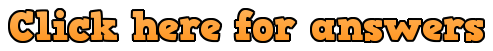# Types of Triangle Textbook AnswersWorkout

Question 1

(a)  Right angle

(b)  Scalene

(c)  Isosceles

(d)  Equilateral

(e)  Isosceles

(f)  Scalene

Question 2

Isosceles right angle triangle … or … Right angled isosceles triangle

Question 3:  A triangle like Q1(a)

Question 4:  A triangle like Q1(c)

Question 5:  A triangle like Q1(b)

Question 6:  A triangle like Q1(d)

Apply

Question 1:  Isosceles

Question 2:  Equilateral

Question 3:

(a)  False

(b)  True

(c)  True

Question 4:

The lengths of the two shorter sides need to add together to be larger than the longest side in order for the sticks to make a triangle.

or… the two shorter sticks would not meet if they were joined to the ends of the 10cm stick.

Question 5:

The angles in a triangle add together to equal 180°. If there is a right angle (90°) and an obtuse (greater than 90°), then just those two angles add together to greater than 180°.